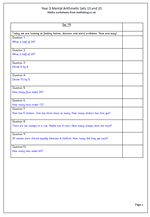# Year 3 Mental Arithmetic: Sets 19 and 20Today I have published another two sets of 10 mental arithmetic questions suitable for Year 3 children (7 to 8 years old). This week I have concentrated on;

a. finding halves of even 2-digit numbers

b. dividing by 4 and 5

c. simple word problems.

Year 3 is an important stage in children’s mathematical development as there is a move away from the concrete to the more abstract. It is a year when it is expected that tables are starting to be learnt, but it is vital that they understand what they mean. Questions involving tables can be asked in a wide variety of ways, including the equivalent division calculation.

Don’t forget that these questions can be answered on paper, by calling out, or by using number cards or number fans. Each way has its own advantages.

All the mental arithmetic papers can be found in the ‘Know Number Facts’ category of the Year 3 worksheets.

Year 3 mental arithmetic sets 19 and 20